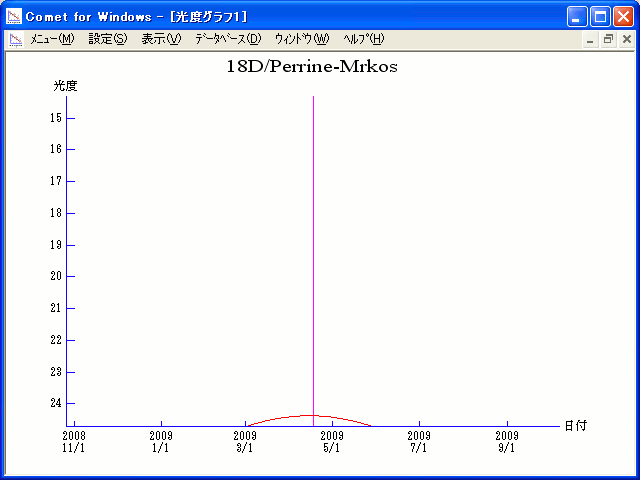# 18D/Perrine-Mrkos (2009)###Orbital Elements

```Perturbed ephemeris below is based on elements from NK 573.

Epoch = 2454920.50000 TT   T = 2454938.75931 TT
Peri. =  156.80416         a =     3.9442172 AU
Node  =  238.06666 J2000.0 e =     0.5851811
Incl. =   16.86718         q =     1.6361359 AU
```

###Magnitudes Graph

```        m1 = 18.0 + 5 log d + 20.0 log r
```##### The orbital elements are calculated using the Minor Planet & Comet Ephemeris Service. The magnitudes graphs are made with Comet for Windows.